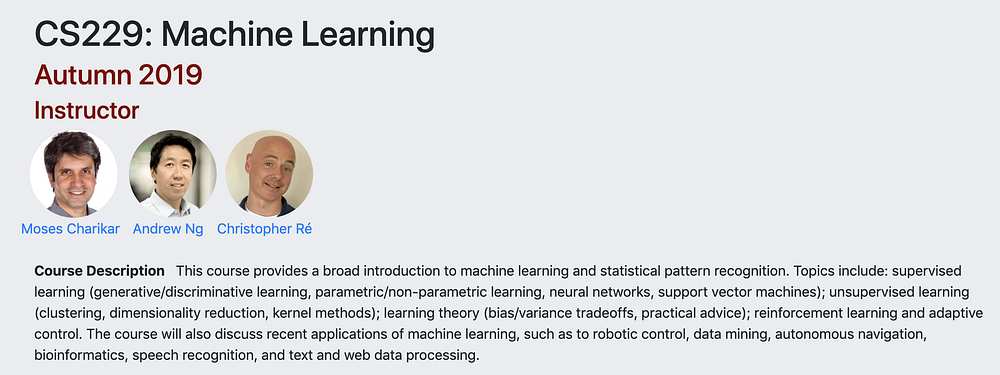# Summary of algorithms in Stanford Machine Learning (CS229) Part III

Source: Deep Learning on Medium## Reinforcement learning in general

In supervised learning, we try to make output mimic label y given input x. In this setting, the labels gave an unambiguous “right answer” for each input x. In reinforcement learning, since it involves a sequence of decision making, it hard to provide this type of explicit supervision. Instead we provide a reward function which indicates to the learning algorithm when it is doing well or poorly. You can think of our learning algorithm as an agent and it interacts with the environment. It knows its state and learns the action at each state, it can get reward from the environment. The end goal of our learning algorithm is to maximize expected cumulative reward.

## Markov decision process (MDP)

MDP is defined by:

• A set of states S. S can be finite or infinite
• A set of actions A. A can be finite or infinite
• Psa, the state transition probabilities. Psa gives the distribution over what states we will transition to if we take action a in state s
• r is called discount factor which is in the range of 0 and 1
• reward function R, which takes state or state and action and outputs a real value

The dynamics of an MDP is that we start in some state s0, choose action a0, based on Ps0a0, we transit to state s1 and so on. Depending on our reward function, the total payoff is: